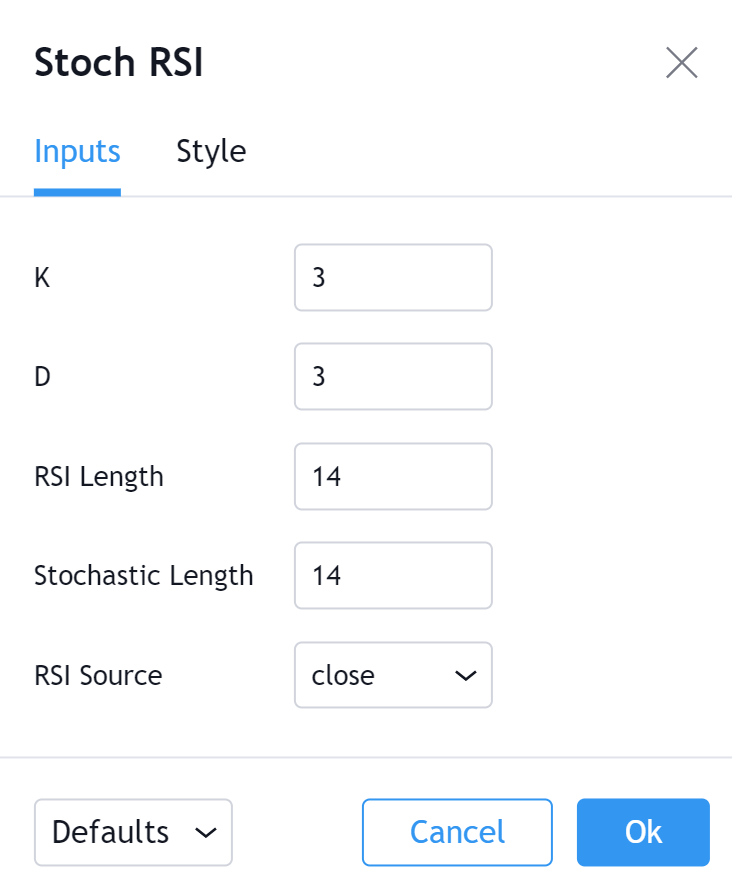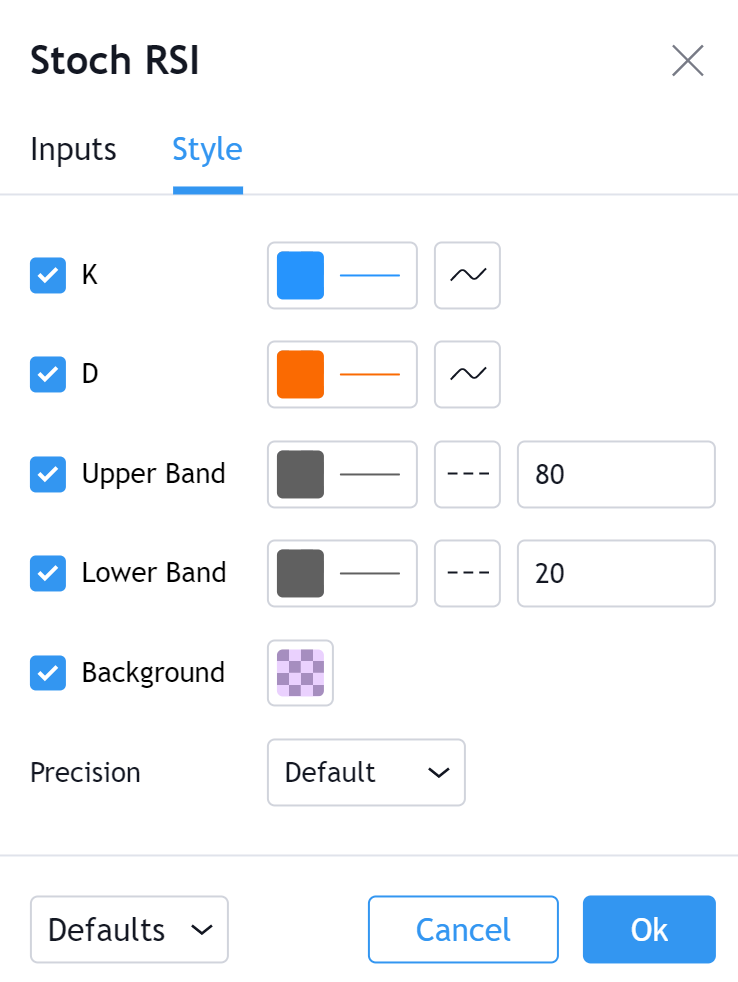# 随机相对强弱指数 (STOCH RSI)

#### `Stoch RSI = (RSI - Lowest Low RSI) / (Highest High RSI - Lowest Low RSI)`以下是一些大致的基准水平：`14 Day Stoch RSI = 1 when RSI is at its highest level in 14 Days. 14 Day Stoch RSI = .8 when RSI is near the high of its 14 Day high/low range. 14 Day Stoch RSI = .5 when RSI is in the middle of its 14 Day high/low range. 14 Day Stoch RSI = .2 when RSI is near the low of its 14 Day high/low range. 14 Day Stoch RSI = 0 when RSI is at its lowest level in 14 Days.`K

D

% D = 价格与先前价格平均值之间的偏差百分比（动量）。 用于计算 %D 的时间段。 3 是默认值。

RSI长度

RSI 来源K

D